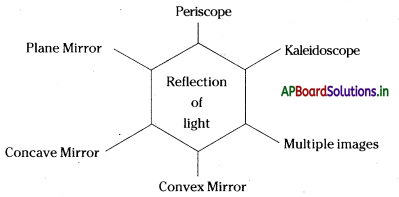# AP Board 7th Class Science Notes Chapter 9 Reflection of Light

Students can go through AP State Board 7th Class Science Notes Chapter 9 Reflection of Light to understand and remember the concept easily.

## AP State Board Syllabus 7th Class Science Notes Chapter 9 Reflection of Light

→ Light coming from a narrow slit or small hole looks like a ray of light.

→ The ray of light falling on the mirror is called the incident ray.

→ The ray of light returning from the mirror is called the reflected ray.

→ The angle between the normal and the incident ray is called the angle of incidence ∠i

→ The angle between the normal and the reflected ray is called the angle of reflection ∠r

→ Periscope is used to view objects outside the room through the window while hiding oneself in the room.

→ In-plane mirror there will be a lateral inversion in the image formed for an object.

→ We get multiple images of an object placed in between the plane mirrors arranged at an angle to each other.

→ The image formed by a rearview mirror is smaller than the image in a plane mirror.

→ If the reflecting surface of a mirror is concave it is called a concave mirror.

→ If the reflecting surface is convex, then it is called a convex mirror.

→ The image that can be obtained on a screen is called a Real image.→ The image that cannot be obtained on a screen is called a virtual image.

→ Reflection from a smooth surface like that of a mirror is called regular reflection.

→ Reflection from a rough or irregular surface is called irregular reflection or diffused reflection.

→ Light changes its direction when it is obstructed by any object. This phenomenon is called reflection.

→ The angle of incidence is equal to an angle of reflection. We denote the angle of incidence with ∠i and the angle of reflection with ∠r.

→ The measure of ∠i = measure of ∠r. (for a plane mirror)

→ In the image formed by a mirror, the right of the object appears as left, and the left of the object appears as right. This is called Lateral Inversion.

→ The distance from the object to a plane mirror is equal to the distance of the image from the mirror.

→ Irrespective of the size of the plane mirror, the size of the image in the mirror is equal to the size of the object.

→ Any object which is far away from us looks smaller in size. In the same way, its image in the plane mirror will also look smaller.

→ The plane mirror forms a single image. If we want to form multiple images two plane mirrors need to be kept at an angle.

→ The number of images increases when we reduce the angle between two mirrors.

→ In a rearview mirror (convex mirror) we get diminished Usage of the object.→ In a mirror which is used by a Dentist (concave mirror), we get an enlarged image of the object

→ The image that can be obtained on a screen is called a Real Image.

→ The image that can’t be obtained on a screen but can be viewed in the mirror is called a Virtual Image.

→ Torches, headlights of vehicles have concave mirrors behind the bulb for reflection.

→ Reflection from a smooth surface is called regular reflection.

→ Reflection from a rough surface is called an irregular reflection.

→ Incident Ray: A ray of light that hits a surface

→ Reflected Ray: The ray represents the light refracted by the surface

→ Normal: Usual

→ The angle of incidence ∠i: The angle made by the incident ray with a normal

→ The angle of reflection ∠r: The angle made by the retracted with normal

→ Periscope: Observation from a concealed position

→ Lateral inversion: The image formed in a flat mirror

→ Kaleidoscope: A cylinder with a mirror.

→ Rearview mirror: Mirror used in vehicles to see the vehicles behind them.

→ Spherical mirror: Mirror in the shape of a circle

→ Convex mirror: Curves outward towards a light source

→ Concave mirror: Curves inward to away the light source

→ Real Image: Image which can be seen with a help of source

→ Virtual image: Image which cannot be seen with a help of source→ Regular Reflection: Reflection occurring on a smooth reflecting surface

→ Irregular Reflection: Reflection from a rough or irregular surface.→ Christian Huygens:

• Huygens was born in 1629. He propounded the wave theory of light.
• He explained the transverse nature of light by constructing a wavefront.
• He died in 1695.